— 创世纪—数理统计

# 1. 正态分布，熟悉的陌生人

$$\displaystyle f(x)=\frac{1}{\sqrt{2\pi}\sigma}e^{-\frac{{(x-\mu})^2}{2\sigma^2}}$$

$$\displaystyle f(x)=\frac{1}{\sqrt{2\pi}}e^{-\frac{x^2}{2}}$$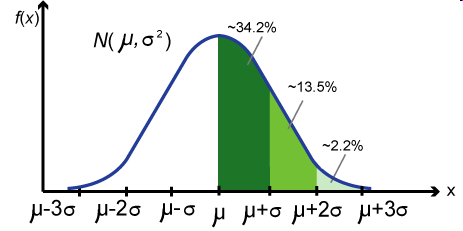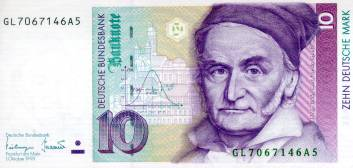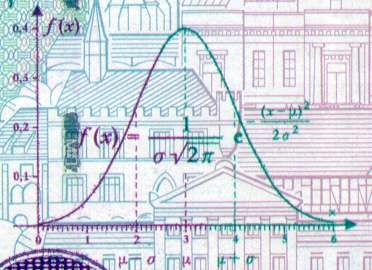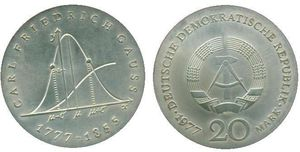# 2.邂逅，正态曲线的首次发现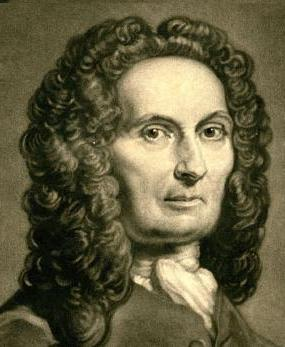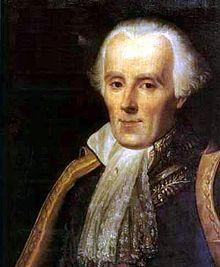$$2npq b(n, p, np)$$

$$\displaystyle n! \approx \sqrt{2\pi n} \left(\frac{n}{e}\right)^n .$$

$$b(n, \frac{1}{2}, i) = \binom{n}{i}\left(\frac{1}{2}\right)^n$$

$$\displaystyle b\left(\frac{n}{2}\right) \approx \sqrt{\frac{2}{\pi n}},$$

$$\displaystyle \frac{b\left(\frac{n}{2}+d\right)}{b\left(\frac{n}{2}\right)} \approx e^{-\frac{2d^2}{n}},$$

$$\displaystyle b\left(\frac{n}{2}+d\right) \approx \frac{2}{\sqrt{2 \pi n}}e^{-\frac{2d^2}{n}}.$$

\begin{eqnarray} \begin{array}{lll} \displaystyle P\left(\left|\frac{X}{n} – \frac{1}{2}\right| \le \frac{c}{\sqrt{n}}\right) & = & \displaystyle \sum_{-c\sqrt{n} \le i \le c\sqrt{n}}b\left(\frac{n}{2}+i\right) \\ & \approx & \displaystyle \sum_{-c\sqrt{n} \le i \le c\sqrt{n}} \frac{2}{\sqrt{2 \pi n}}e^{-\frac{2i^2}{n}} \\ & = & \displaystyle \sum_{-2c \le \frac{2i}{\sqrt{n}} \le 2c} \frac{1}{\sqrt{2 \pi}}e^{-\frac{1}{2}(\frac{2i}{\sqrt{n}})^2} \frac{2}{\sqrt{n}} \\ & \approx &\displaystyle \int_{-2c}^{2c} \frac{1}{\sqrt{2\pi}} e^{-x^2/2} dx . \end{array} \end{eqnarray}

**[棣莫弗-拉普拉斯中心极限定理]**设随机变量 $$X_n (n=1,2,\cdots)$$ 服从参数为 $$n,p$$ 的二项分布，则对任意的 $$x$$, 恒有

$$\lim_{n\rightarrow\infty}P\left( \frac{X_n – np}{\sqrt{np(1-p)}} \le x \right) = \int_{-\infty}^x \frac{1}{\sqrt{2\pi}} e^{\frac{-t^2}{2}}dt .$$

# 3. 最小二乘法，数据分析的瑞士军刀

• 土星和木星是太阳系中的大行星，由于相互吸引对各自的运动轨道产生了影响，许多大数学家，包括欧拉和拉普拉斯都在基于长期积累的天文观测数据计算土星和木星的运行轨道。
• 勒让德承担了一个政府给的重要任务，测量通过巴黎的子午线的长度。
• 海上航行经纬度的定位。主要是通过对恒星和月面上的一些定点的观测来确定经纬度。

$$y = \beta_0 + \beta_1x_1 + \cdots + \beta_px_p$$

\begin{eqnarray} \left\{ \begin{array}{lll} y_1 = \beta_0 + \beta_1x_{11} + \cdots + \beta_px_{p1} \\ y_2 = \beta_0 + \beta_1x_{12} + \cdots + \beta_px_{p2} \\ \vdots \\ y_n = \beta_0 + \beta_1x_{1n} + \cdots + \beta_px_{pn} . \end{array} \right. \end{eqnarray}

\begin{eqnarray} \label{least-square-error} \begin{array}{lll} \hat{\boldsymbol\beta}& = & \displaystyle \arg\min_{\boldsymbol {\beta}} \sum_{i=1}^n e_i^2 \\ & = & \displaystyle \arg\min_{\boldsymbol\beta} \sum_{i=1}^n\Bigl[y_i – (\beta_0 + \beta_1x_{1i} + \cdots + \beta_px_{pi})\Bigr]^2 . \end{array} \end{eqnarray}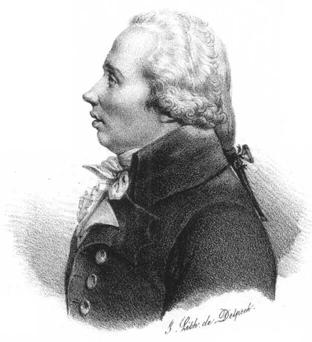1. 最小二乘法使得误差平方和最小，并在各个方程的误差之间建立了一种平衡，从而防止某一个极端误差取得支配地位；
2. 计算中只要求偏导后求解线性方程组，计算过程明确便捷；
3. 最小二乘法可以导出算术平均值作为估计值。

$$L(\theta) = \sum_{i=1}^n e_i^2 = \sum_{i=1}^n (x_i – \theta)^2$$

# 4. 众里寻她千百度,误差分布曲线的确立

1. 观测数据存在误差
2. 误差是对称分布的;
3. 大的误差出现频率低，小的误差出现频率高。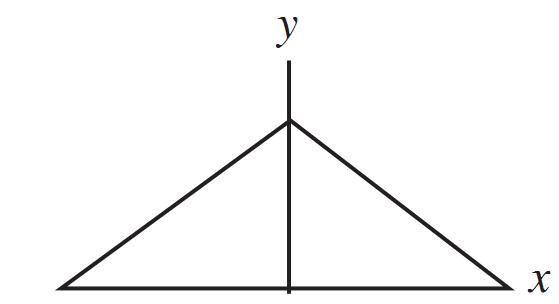$$P(|\overline{e}| < x) \ge P(|e_i|<x) .$$

$$-f'(x) = mf(x)$$

\begin{equation} \label{laplace-error-curve} f(x) = \frac{m}{2} e^{-m|x|} . \end{equation}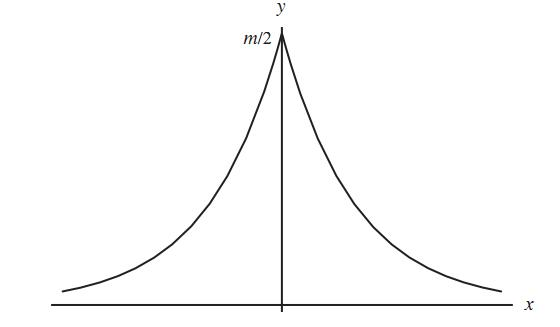\begin{align*} L(\theta) & = L(\theta;x_1,\cdots,x_n)=f(e_1)\cdots f(e_n) \\ & = f(x_1-\theta)\cdots f(x_n-\theta) \end{align*}

$$\hat{\theta}= \arg\max_{\theta} L(\theta) .$$

$$f(x)=\frac{1}{\sqrt{2\pi}\sigma}e^{-\frac{x^2}{2\sigma^2}}$$

17、18世纪科学界流行的做法，是尽可能从某种简单明了的准则(first principle)出发进行逻辑推导。高斯设定了准则“最大似然估计应该导出优良的算术平均”，并导出了误差服从正态分布，推导的形式上非常简洁优美。但是高斯给的准则在逻辑上并不足以让人完全信服，因为算术平均的优良性当时更多的是一个经验直觉，缺乏严格的理论支持。高斯的推导存在循环论证的味道：因为算术平均是优良的，推出误差必须服从正态分布；反过来，又基于正态分布推导出最小二乘法和算术平均，来说明最小二乘法和算术平均的优良性。这陷入了一个鸡生蛋蛋生鸡的怪圈，逻辑上算术平均的优良性到底有没有自行成立的理由呢？

Many years ago I called the Laplace-Gaussian curve the normal curve, which name, while it avoids an international question of priority, has the disadvantage of leading people to believe that all other distributions of frequency are in one sense or another “abnormal”.

—Karl Pearson (1920)

# 5. 曲径通幽处，禅房花木深

## 5.1 高斯(1809)的推导

\begin{align*} L(\theta) &= L(\theta;x_1,\cdots,x_n)=f(e_1)\cdots f(e_n) \\ & = f(x_1-\theta)\cdots f(x_n-\theta) \end{align*}

$$\frac{d \log L(\theta)}{d \theta} = 0$$

$$\sum_{i=1}^n \frac{f'(x_i-\theta)}{f(x_i-\theta)} = 0$$

$$g(x) = \frac{f'(x)}{f(x)}$$,

$$\sum_{i=1}^n g(x_i-\theta) = 0$$

\begin{equation} \sum_{i=1}^n g(x_i-\bar{x}) = 0 \quad  (1) \end{equation}

(1)式中取 $$n=2$$, 有

$$g(x_1-\bar{x}) + g(x_2-\bar{x}) = 0$$

$$g(-x) = -g(x)$$

(1)式中再取 $$n=m+1$$, 并且要求 $$x_1=\cdots=x_m=-x, x_{m+1} = mx$$, 则有 $$\bar{x} = 0$$, 并且

$$\sum_{i=1}^n g(x_i-\bar{x}) = mg(-x) + g(mx)$$

$$g(mx) = mg(x)$$

$$f(x) = Me^{cx^2}$$

$$N(0,\sigma^2)$$

## 5.2 赫歇尔(1850)和麦克斯韦(1860) 的推导

$$p(x,y)$$,赫歇尔设置了两个准则：

1. $$x$$ 轴和 $$y$$ 轴的误差是相互独立的，即随机误差在正交的方向上相互独立
2. 误差的概率分布在空间上具有旋转对称性，即误差的概率分布和角度没有关系

$$p(x,y) = f(x) * f(y)$$

$$p(x,y) = p(rcos\theta, rsin\theta) = g(r,\theta)$$

$$f(x)f(y) = g(r) = g(\sqrt{x^2+y^2})$$

$$y=0$$, 得到 $$g(x) = f(x)f(0)$$, 所以上式可以转换为

$$\log\left[\frac{f(x)}{f(0)}\right] + \log\left[\frac{f(y)}{f(0)}\right]= \log\left[\frac{f(\sqrt{x^2+y^2})}{f(0)}\right]$$

$$\log\left[\frac{f(x)}{f(0)}\right] = h(x)$$, 则有

$$h(x) + h(y) = h(\sqrt{x^2+y^2})$$

$$f(x) = \sqrt{\frac{\alpha}{\pi}} e^{-\alpha x^2}$$

$$f(x)$$ 就是正态分布 $$N(0, 1/\sqrt{2\alpha)}$$， 从而 $$p(x,y)$$ 就是标准二维正态

$$p(x,y) = \frac{\alpha}{\pi} e^{-\alpha (x^2+y^2)}.$$

1860 年，伟大的物理学家麦克斯韦在考虑气体分子的运动速度分布的时候，在三维空间中基于类似的准则推导出了气体分子运动的分布是正态分布 $$\rho(v_x,v_y,v_z)\propto\exp\{-\alpha(v_x^2+v_y^2+v_z^2)\}$$。这就是著名的麦克斯韦分子速率分布定律。大家还记得我们在普通物理中学过的麦克斯韦-波尔兹曼气体速率分布定律吗？

\begin{eqnarray} \label{maxwell} \begin{array}{lll} F(v) & = & \displaystyle \left(\frac{m}{2\pi kT}\right)^{3/2} e^{-\frac{mv^2}{2kT}} \\ & = & \displaystyle \left(\frac{m}{2\pi kT}\right)^{1/2} e^{-\frac{mv_x^2}{2kT}} \times \left(\frac{m}{2\pi kT}\right)^{1/2} e^{-\frac{mv_y^2}{2kT}} \times \left(\frac{m}{2\pi kT}\right)^{1/2} e^{-\frac{mv_z^2}{2kT}} . \end{array} \end{eqnarray}

## 5.3 兰登(1941)的推导

1. 随机噪声具有稳定的分布模式
2. 累加一个微小的随机噪声，不改变其稳定的分布模式，只改变分布的层级(用方差度量)

$$X \sim p(x;\sigma^2), \epsilon \sim q(e), X’= X+\epsilon$$

$$X’ \sim p(x;\sigma^2 + var(\epsilon))$$

$$f(x’) = \int p(x’-e; \sigma^2)q(e)de$$

$$p(x’-e; \sigma^2)$$$$x’$$处做泰勒级数展开(为了方便，展开后把自变量由$$x’$$ 替换为 $$x$$)， 上式可以展开为

$$f(x) = p(x; \sigma^2) – \frac{\partial p(x; \sigma^2)}{\partial x} \int eq(e)de +\frac{1}{2} \frac{\partial^2 p(x; \sigma^2)}{\partial x^2} \int e^2q(e)de + \cdots$$

$$p(x; \sigma^2)$$简记为$$p$$,则有

$$f(x) = p – \frac{\partial p}{\partial x} \overline{\epsilon} +\frac{1}{2} \frac{\partial^2 p}{\partial x^2}\overline{\epsilon^2} + o(\overline{\epsilon^2})$$

\begin{equation} \label{landon-x} f(x) = p + \frac{1}{2} \frac{\partial^2 p}{\partial x^2}\overline{\epsilon^2} + o(\overline{\epsilon^2}) \quad (2) \end{equation}

\begin{equation} \label{landon-sigma} f(x) = p + \frac{\partial p}{\partial \sigma^2}\overline{\epsilon^2} + o(\overline{\epsilon^2})  \quad (3) \end{equation}

$$\frac{1}{2} \frac{\partial^2 p}{\partial x^2} = \frac{\partial p}{\partial \sigma^2}$$

$$p(x; \sigma^2) = \frac{1}{\sqrt{2\pi}\sigma}e^{-\frac{x^2}{2\sigma^2}}$$

## 5.4 基于最大熵的推导

$$H(p) = -\int p(x)\log p(x) dx$$

$$\int p(x) \log \frac{q(x)}{p(x)} dx \le \int p(x) (\frac{q(x)}{p(x)} – 1) dx = \int q(x) dx – \int p(x) dx = 0$$

$$\int p(x) \log \frac{q(x)}{p(x)} dx = \int p(x) \log\frac{1}{p(x)}dx + \int p(x) \log q(x) dx \le 0$$

\begin{equation} H(p) \le -\int p(x) \log q(x) dx \end{equation}

\begin{eqnarray} \begin{array}{lll} H(p) & \le &\displaystyle – \int p(x) \log \left\{ \frac{1}{\sqrt{2\pi}\sigma} e^{-\frac{{(x-\mu})^2}{2\sigma^2}}\right\} dx \\ & = & \displaystyle \int p(x) \left\{ \frac{(x-\mu)^2}{2\sigma^2} + \log \sqrt{2\pi}\sigma \right\} dx \\ & = & \displaystyle \frac{1}{2\sigma^2} \int p(x)(x-\mu)^2 dx + \log \sqrt{2\pi}\sigma \end{array} \end{eqnarray}

$$\int p(x) (x-\mu)^2 dx = \sigma^2$$

$$H(p) \le \frac{1}{2\sigma^2}\sigma^2 + \log \sqrt{2\pi}\sigma = \frac{1}{2} + \log \sqrt{2\pi}\sigma$$

Physicists believe that the Gaussian law has been proved in mathematics while mathematicians think that it was experimentally established in physics.

—— Henri Poincaré